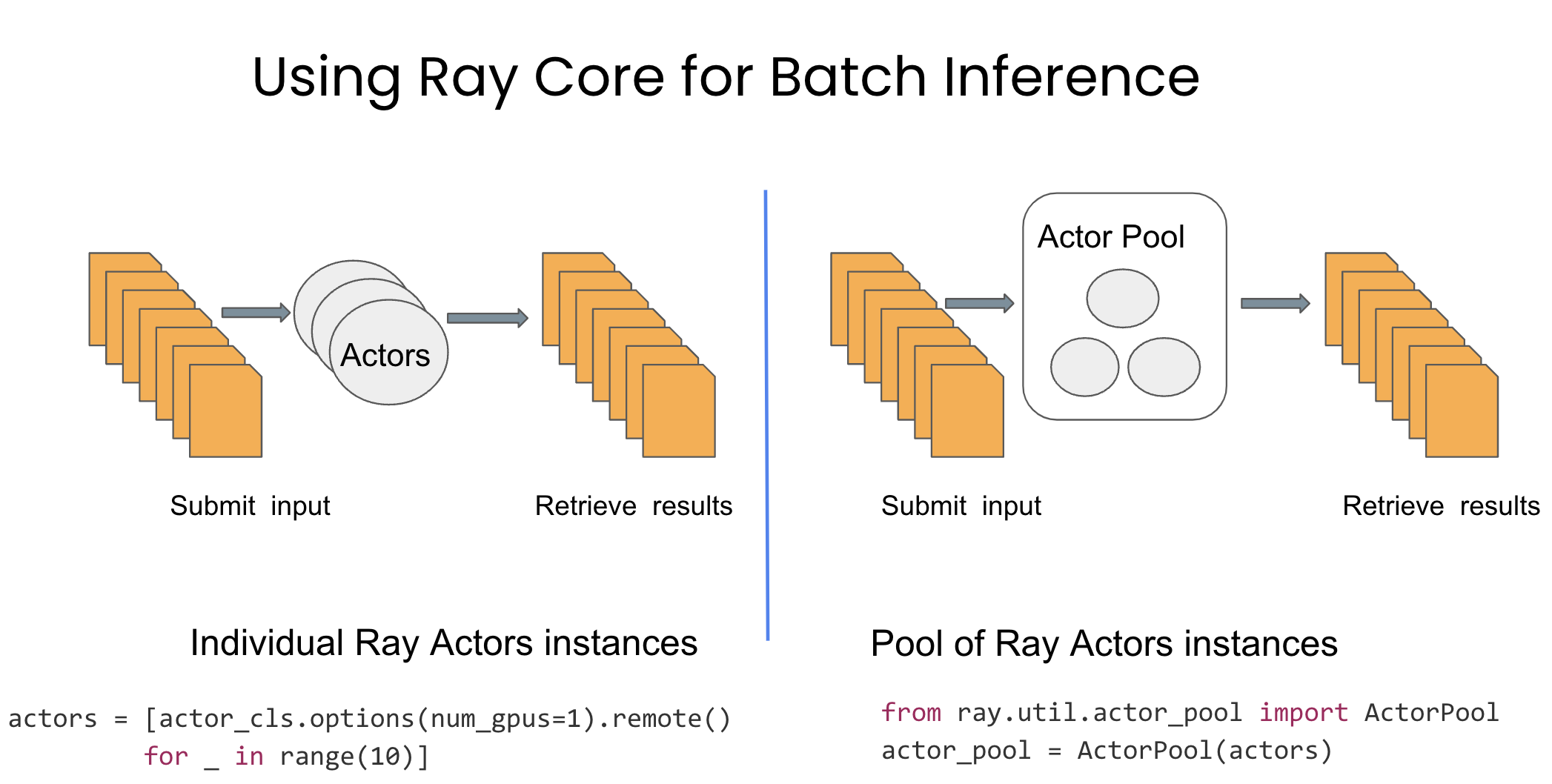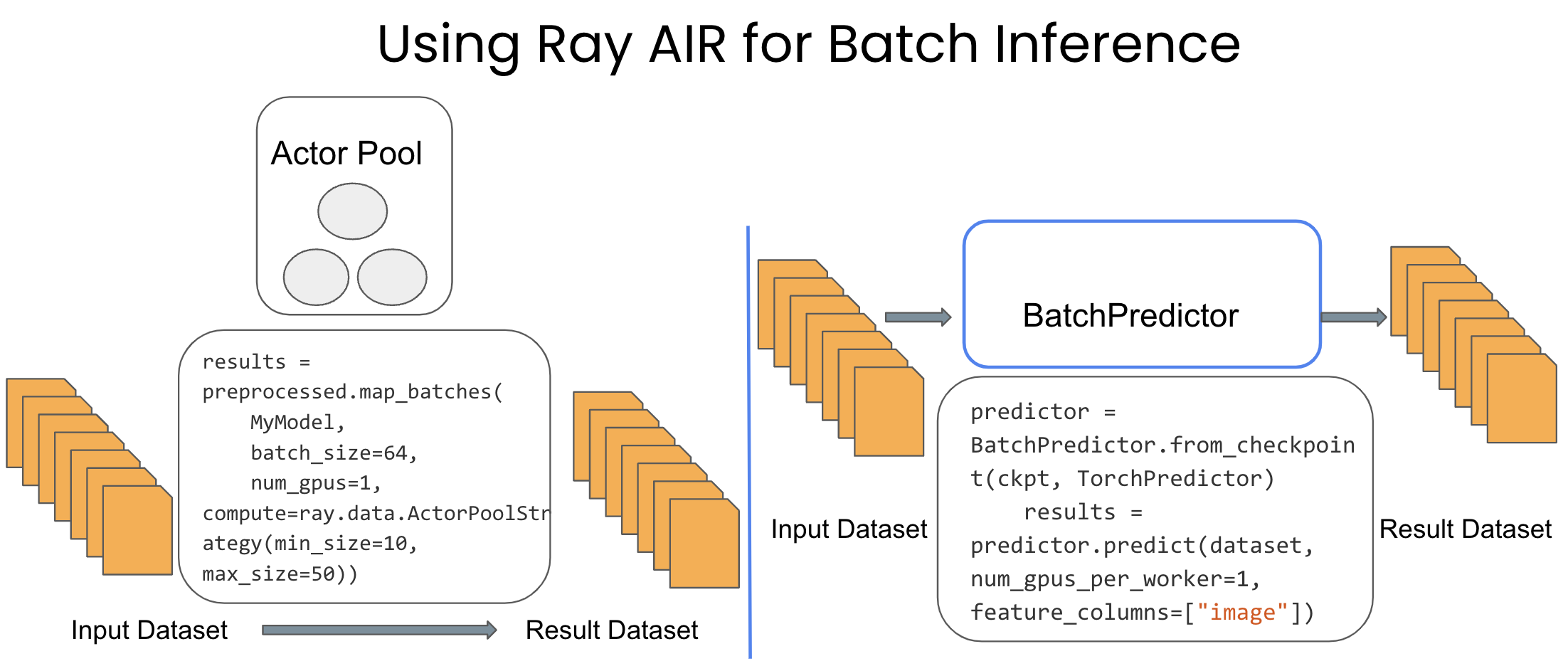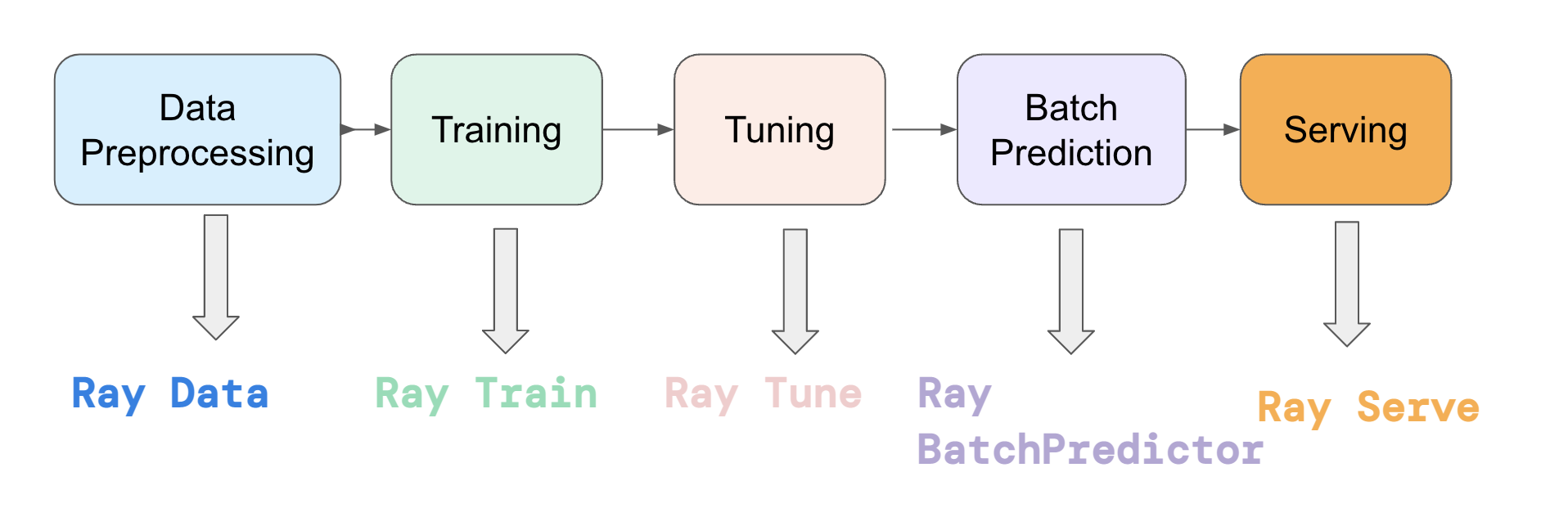# Model Batch Inference in Ray: Actors, ActorPool, and Datasets

This blog covers four methods of batch inference (distributed model evaluation) in Ray: from low-level using Ray Actors, to high-level using Ray AIR libraries. We'll see how low-level Ray APIs allow you to control exactly "How" Ray is executing computations, while Ray's libraries enable you to specify just the "What" --- for a more out-of-the-box scalable experience.Starting with a local Python class defining your trained model, Ray makes it easy to parallelize inference over the model using any of these ways. In the next sections, we'll walk through examples of how to perform parallel model inference on an NYC taxi data model in Ray 2.0.

Batch inference refers to generating model predictions over a set of input observations. The model could be a regression model, neural network, or simply a Python function. When the model is expensive or the data to be evaluated is large, batch inference can benefit from scaling with Ray. Ray is commonly used for parallelizing batch inference jobs on single machines as well as clusters with thousands of GPUs.

To set up distributed batch inference in any kind of system, the following steps are needed:

1. Create a number of replicas of your model. In Ray, these replicas are represented as Actors (i.e., stateful  processes) that can be assigned to GPUs and hold instantiated model objects.

2. Feed data into these model replicas in parallel, and retrieve inference results.

Let's dive into some examples: we'll start with the low-level ones to build an understanding of "How" Ray executes computations, before showing the higher-level APIs.

## LinkScalable Batch inference in Ray Core

Ray's Actor API is a natural primitive for batch inference. We'll see first how to use Actors directly to distribute batch inference. To simplify the task of dispatching work to a large number of Actors of the same kind, Ray also provides an ActorPool utility.## LinkStarting from Scratch with Ray Actors

For all these examples, let's assume a large number of records in S3 at `s3://air-example-data/ursa-labs-taxi-data/ `that we want to run a custom model against for inference. We can start by defining a simple "pretrained" model and an Ray Actor that can be constructed from a model reference:

``````1
2
3
4
5
6
7
8
9
10
11
12
13
14
15
16
17
18
19
20
21
22
23
24
25
import pandas as pd
import pyarrow.parquet as pq
import ray

# A fake model that predicts whether tips were given based on
# the number of passengers in the taxi cab.
def model(batch: pd.DataFrame) -> pd.DataFrame:
# Give a tip if 2 or more passengers.
predict = batch["passenger_count"] >= 2
return pd.DataFrame({"score": predict})
return model

@ray.remote
class NYCBatchPredictor:
def __init__(self, model):
self.model = model

def predict(self, split_path: str):
# read each split and convert to pandas

# do the inference with our model and return the result
result = self.model(df)
return result``````

To parallelize this with Ray, we put the model into the Ray object store, and then launch a number of our predictor actors as follows:

``````1
2
3
model_ref = ray.put(model)
actors = [NYCBatchPredictor.remote(model_ref) for _ in range(5)]``````

Ray automatically fetches and de-references the `model_ref` argument passed to the actor constructor, so the NYCPredictorActor sees a materialized model object instead of a reference to it. Next we need to define our input files:

``````1
2
3
input_splits = [f"s3://anonymous@air-example-data/ursa-labs-taxi-data/downsampled_2009_full_year_data.parquet"
f"/fe41422b01c04169af2a65a83b753e0f_{i:06d}.parquet"
for i in range(12) ]``````

We can dispatch and retrieve results to these actors using a` ray.wait` loop. Basically, we want to keep a certain backlog of tasks "in-flight" to the actors, retrieving ready results and sending new tasks to actors as they finish with previous work:

``````1
2
3
4
5
6
7
8
9
10
11
12
13
14
15
16
17
18
19
def process_result(r):
print(f"Predictions dataframe size: {len(r)} | Total score for tips: {r['score'].sum()}")

idle_actors = actors.copy()
future_to_actor = {}
while input_splits:
if idle_actors:
actor = idle_actors.pop()
future = actor.predict.remote(input_splits.pop())
future_to_actor[future] = actor
else:
actor = future_to_actor.pop(future)
idle_actors.append(actor)
process_result(ray.get(future))

# Process any leftover results at the end.
for future in future_to_actor:
process_result(ray.get(future))
``````

Running this produces output like:

``````1
2
3
4
5
6
7
8
9
10
11
12
Predictions dataframe size: 136999 | Total score for tips: 45142
Predictions dataframe size: 136394 | Total score for tips: 43234
Predictions dataframe size: 141981 | Total score for tips: 45188
Predictions dataframe size: 148108 | Total score for tips: 47713
Predictions dataframe size: 143087 | Total score for tips: 45510
Predictions dataframe size: 144014 | Total score for tips: 45175
Predictions dataframe size: 133932 | Total score for tips: 42175
Predictions dataframe size: 145976 | Total score for tips: 48036
Predictions dataframe size: 142893 | Total score for tips: 46112
Predictions dataframe size: 156198 | Total score for tips: 49909
Predictions dataframe size: 139985 | Total score for tips: 44138
Predictions dataframe size: 141062 | Total score for tips: 46360``````

The above example implements (1) distributed dispatch of tasks to a large number of actors, and (2) processing results from the actors and dispatching new work in a streaming way. It's not too many lines of code for a distributed program, but we can do better! Ray provides an ActorPool utility that makes this a lot easier.

### LinkUsing ActorPool as a utility library for batch inference

Suppose we have our list of actors created. We can wrap it in an ActorPool class as follows:

``````1
2
from ray.util.actor_pool import ActorPool
actor_pool = ActorPool(actors)
``````

Then, to process our data, all we need to do is call `actor_pool.map.` Actually, we'll use `.map_unordered` for slightly better efficiency as we don't care about the order of results:

``````1
2
3
4
5
def actor_call(actor, data):
return actor.predict.remote(data)

for result in actor_pool.map_unordered(actor_call, input_splits):
process_result(result)
``````

The above snippet does exactly the same logic as the original loop, just hiding the tedious futures management in a convenient utility class. You can check out the source code of `ActorPool` to see the familiar` ray.wait` primitive it is using under the hood.

There are a few unoptimized aspects of the above code to point out. First, we are dispatching file splits one at a time, which may be inefficient if the splits are too small (e.g., on GPUs) – or cause OutOfMemory errors if the splits are too large. Furthermore, you may want to pipeline the task submission (have multiple tasks in flight to an actor at once), and parallelize the data fetching and any necessary preprocessing.

While we could implement these optimizations on top of the example code above, perhaps using Ray tasks to parallelize data fetching, for example, Ray's Datasets and BatchPredictor libraries have these optimizations built-in, as well as other features such as dynamic autoscaling of the actor pool used for the computation.

The upshot or takeaways from above is as follows:

1. You can build distributed batch inference using Ray Actors and ActorPool.

2. You can control exactly how  Ray is executing your code.

3. You understand how Ray works under the hood with respect to Ray's core primitives, such as tasks, actors, objects, and `ray.wait`.

However, all these optimizations force you to implement common performance optimizations yourself, giving you the control yet demanding internal knowledge of Ray.

But there is a better way to instruct Ray what it is that you want done, via a set of composable Ray AI Runtime (AIR) APIs, without deep knowledge of Ray core’s primitive. We discuss our next approach with Ray AIR.

## LinkScalable batch inference in Ray AIR

It can be tricky and time-consuming to implement and test optimizations for a distributed program. The goal of Ray AI Runtime is to provide optimized high-level libraries for common use cases such as batch inference, handling:

1. Dynamic autoscaling of the actor pool.

2. Automatic batching and pipelining of data.

3. Parallelizing data fetching and preprocessing.## LinkUsing Ray Datasets to parallelize preprocessing and inference for batch inference

Among other common components (training, tuning, scoring, and serving) in your machine learning pipeline, Ray AIR provides a Datasets library for easy loading and preprocessing of feature data in Ray.As a first step, we can use `ray.data.read_parquet` here to load the data as a Ray Dataset:

``````1
2
3

# -> Dataset(num_blocks=12, num_rows=1710629, schema={vendor_id: string, pickup_at: timestamp[us], dropoff_at: timestamp[us], passenger_count: int8, trip_distance: float, pickup_longitude: float, pickup_latitude: float, rate_code_id: null, store_and_fwd_flag: string, dropoff_longitude: float, dropoff_latitude: float, payment_type: string, fare_amount: float, extra: float, mta_tax: float, tip_amount: float, tolls_amount: float, total_amount: float})``````

We can also do preprocessing on our Dataset using simple transformation APIs like map_batches. Here's an example of shifting the `num_passengers` field by 1.0 via a Dataset transform:

``````1
2
3
4
5
6
# Define our preprocessing function
def preprocess(batch):
batch["passenger_count"] -= 1.0
return batch

ds = ds.map_batches(preprocess)
``````

To proceed, let's define our model class to use (note that we define the` __call__` method of the class to make it a callable class and specify the target method):

``````1
2
3
4
5
6
7
class CallableCls:
def __init__(self, model):
self.model = model

def __call__(self, batch):
result = self.model(batch)
return result``````

Finally, we can use the Dataset `map_batches() `function to apply our model to our Dataset in parallel. We can specify the batch size to pass to the model, any GPU resources, as well as autoscaling options for the actor pool Datasets is going to use under the hood.

``````1
2
3
4
5
6
7
results = ds.map_batches(
CallableCls,
num_gpus=0,
batch_size=1024,
compute=ray.data.ActorPoolStrategy(min_size=1, max_size=5),
fn_constructor_args=(model_ref,))
# -> Dataset(num_blocks=12, num_rows=1710629, schema={score: bool})``````

Compared to the original version, the Datasets version has a few advantages. For one, it allows for parallel reading and preprocessing of the source data. Second, the Ray Datasets library manages the autoscaling of the ActorPool used for inference. And lastly, we only declared what we want done, with a set of declarative key-value arguments, rather than how it should be done, avoiding all the cumbersome code we wrote above to instruct Ray how to parallelize and scale.

### LinkUsing Ray AIR's BatchPredictor API for batch inference

Finally, we could use Ray's highest-level API for batch inference: BatchPredictor. BatchPredictor takes a Checkpoint representing the saved model, and allows you to perform inference on an input dataset very similar to the above:

``````1
2
3
4
5
6
7
8
9
10
11
12
13
14
15
16
17
18
19
20
21
22
23
24
25
26
from ray.air import Checkpoint
from ray.data.preprocessors import BatchMapper
from ray.train.predictor import Predictor
from ray.train.batch_predictor import BatchPredictor

# Implement a custom AIR predictor class.
class CustomPredictor(Predictor):
def __init__(self, model):
super().__init__()
self.model = model

def _predict_pandas(self, batch):
return self.model(batch)

@classmethod
def from_checkpoint(cls, checkpoint, **kwargs):
return CustomPredictor(checkpoint.to_dict()["model"])

predictor = BatchPredictor(
checkpoint=Checkpoint.from_dict({"model": model}),
predictor_cls=CustomPredictor,
preprocessor=BatchMapper(preprocess),
)

results = predictor.predict(ds)
# -> Dataset(num_blocks=12, num_rows=1710629, schema={score: bool})``````

Notice the simple and composable APIs that are expressive and instruct Ray what to do: a) preprocess data in batches with a built-in BatchMapper, b) use a given custom class for generating predictions, and c) instantiate a distributed predictor using the given checkpoint data. Internally, Ray implements optimizations such as combining preprocessing and prediction operations in the same Ray task.

All done using Ray AIR’s expressive APIs instructing Ray “What to do.” Refer to the AIR Predictor user guide for the next steps.

We explored four ways to implement scalable batch inference in Ray, using Ray’s low-level primitives and Ray AIR’s high-level expressive APIs. Each way has its merits. If you want to control and dictate how Ray should execute your batch inference then use ray tasks and actors or Actor pools utility. By contrast, if you want Ray to manage your scaling, distribution, and inference at scale, use Ray AIR’s BatchPredictor.

These APIs are layered on top of each other – they all are Ray tasks, actors, and objects under the hood. Pick and choose depending on your needs.

The takeaway here is that Ray Core gives you control over how to do something, putting the onus on implementing inference with Ray primitives yourself and understanding how Ray works under the hood, whereas Ray AIR, with its BatchPredictor, offers automatic scaling, expressive, and intuitive APIs to conduct batch inference at scale. Additionally, the latter offers less code and more brevity.

For data scientists and machine learning practitioners who care more about getting the models to scale for batch inference and worry less about underlying primitives and under-the-hood execution details, Ray AIR is a desirable option.